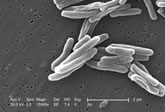# M. Tuberculosis Genes

## Donated on 7/13/2001

Data giving characteristics of each ORF (potential gene) in the M. tuberculosis bacterium. Sequence, homology (similarity to other genes) and structural information, and function (if known) are provided

Relational

Life Science

-

-

-

-

# Dataset Information

The data was collected from several sources, including the Sanger Centre (http://www.sanger.ac.uk/Projects/M_tuberculosis/Gene_list/) and SWISSPROT (http://www.expasy.ch/sprot/sprot-top.html). Structure prediction was made by PROF (http://www.aber.ac.uk/~phiwww/prof/index.html). Homology search was made by PSI-BLAST (http://www.ncbi.nlm.nih.gov/BLAST/). The data is in Datalog format. Missing values are not explicit, but some genes have more relationships than others. Dependencies: M. tuberculosis genes (ORFs) are related to each other by the predicate tb_to_tb_evalue(TBNumber,E-value). They are related to other (SWISSPROT) proteins by the predicate e_val(AccNo,E-value). All the data for a single gene (ORF) is enclosed between delimiters of the form: begin(model(TBNumber)). end(model(TBNumber)). Other Relevant Information: The gene functional classes are in a hierarchy. See http://www.sanger.ac.uk/Projects/M_tuberculosis/Gene_list/. There are two datalog files: tb_data.pl and ecoli_functions.pl 1. tb_functions.pl Lists classes and ORF functions. Lines are of the following form: class([1,0,0,0],"Small-molecule metabolism "). class([1,1,0,0],"Degradation "). class([1,1,1,0],"Carbon compounds "). Arguments are a list of 4 numbers (describing class at the 4 different levels), followed by a string class description. For example, function(tb186,[1,1,1,0],'bglS',"beta-glucosidase"). Arguments are ORF number, list of 4 class numbers, gene name (or null if no gene name) in single quotes, ORF description in double quotes. 2. tb_data.pl Data for each ORF (gene) is delimited by begin(model(X)). end(model(X)). where X is the ORF number. Other predicates are as follows (examples): tb_protein(X). % X is gene number function(2,1,5,0,'gyrA','DNA gyrase subunit A'). % 4 levels of functional hierarchy, gene name, description coding_region(7302,9815). % start,end. integers tb_mol_wt(19934). % integer access(1,e,20). % int (position), {e,i,b}, int (length) access_exposed(1,20). % int (position), int (length) access_intermediate(26,1). % int (position), int (length) access_burried(1,2). % int (position), int (length) access_dist(b,42.8). % {e,i,b}, float (percentage) sec_struc(1,c,23). % int (position), {a,b,c}, int (length) sec_struc_coil(1,23). % int (position), int (length) sec_struc_alpha(1,15). % int (position), int (length) sec_struc_beta(1,6). % int (position), int (length) struc_dist(a,32.1). % {a,b,c}, float (percentage) sec_struc_conf(78.8). % float (confidence) sec_struc_conf_alpha(88.9). % float (confidence) sec_struc_conf_beta(58.0). % float (confidence) sec_struc_conf_coil(77.7). % float (confidence) psi_sequences_found(1,7). % how many found, which iteration psi_sequences_found_again(2,7). % how many found, which iteration psi_sequences_found_new(2,0). % how many found, which iteration amino_acid_ratio(a,11.2). % amino acid letter, float amino_acid_pair_ratio(a,c,0.0). % amino acid letter, amino acid letter, float (out of 1000, ie 2.8 = 0.28%) sequence_length(187). % integer tb_to_tb_evalue(tb3671,1.100000e-01). % ORF number, e-value (double) e_val(p35925,7.0e-59). % SWISSPROT accession no, e-value (double) species(p35925,'streptomyces_coelicolor'). % SWISSPROT acc no, string classification(p35925,bacteria). % SWISSPROT acc no, name mol_wt(p35925,19772). % SWISSPROT acc no, integer keyword(p35925,'hypothetical_protein'). % SWISSPROT acc no, string db_ref(p35925,embl,l27063,g436026,null). % SWISSPROT acc no, db id, primary id, secondary id, status id signalip(c,35,no). % {c,y,s}, int (signal peptide c/y/s score), yes/no signalip(ss,1,34,no). % ss, int, int, yes/no signalip(cleavage,59,60). % cleavage, int/null, int/null hydro_cons(-0.498,-0.474,0.624,3.248,0.278). % double, double, double, double, double gene_name(p41514,'gyrb'). % SWISSPROT acc no, string

Has Missing Values?

No

0 citations
749 views

Ross King